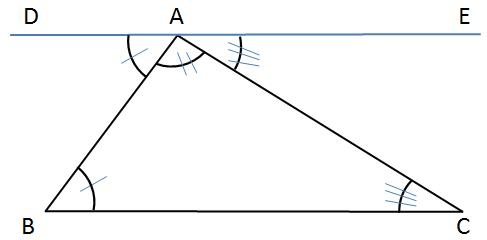Properties of Triangles:

a. Angle Sum Property

Theorem: The sum of the angles of a triangle is 180°.Proof:

Given:$\Delta ABC$

To Prove:$\angle ABC+\angle BCA+\angle CAB=180^{\circ}$

First draw a line$DE$ parallel to$BC (DE \parallel BC)$.$AB \ and\ AC$ are the transversals.

Since$DE \parallel BC$,$\angle ABC=\angle BAD$ (Alternate Angles)……………………….i)

Similarly,$\angle BCA=\angle CAE$ (Alternate Angles)…………………………ii)

Adding i) and ii), we get$\angle ABC+\angle BCA=\angle BAD+\angle CAE$ …………………………iii)

Adding$\angle BAC$ on both sides of iii) we get the following$\angle ABC+\angle BCA+\angle BAC=\angle BAD+\angle CAE+\angle BAC$

Since$\angle BAD+\angle CAE+\angle BAC=180^{\circ}$  (Angles on a straight line)

Hence$\angle ABC+\angle BCA+\angle BAC=180^{\circ}$

or$\angle A+\angle B+\angle C=180^{\circ}$

b. Exterior Angle Property

Theorem: If one side of the triangle is produced, then the exterior angle so formed is equal to the sum of the interior opposite angles.Proof:

To Prove:$\angle ACD=\angle CAB+\angle BAC$

Given: Given:$\Delta ABC$

Extend$BC\ to\ D$. Also draw a like$CE \parallel AB.\ AC\ \&\ BC$ are the transversals.

Therefore,$\angle ACE=\angle BAC$ (Alternate Angles)

Similarly,$\angle DCE=\angle ABC$ (Corresponding Angles)$\angle ACD=\angle ACE+\angle ECD=\angle ABC+\angle BAC$

or$\angle ACD=\angle ABC+\angle BAC$

Hence proved, that if one side of the triangle is produced, then the exterior angle so formed is equal to the sum of the interior opposite angles.